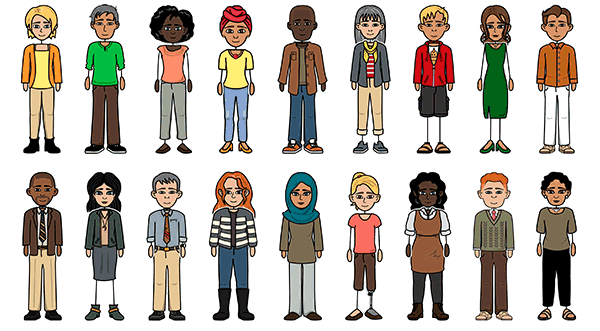# General Chemistry Comic strip

Create a Storyboard#### Storyboard Text

• Tell me, what is it?
• Its Magnesium reacts with Nitrogen gas to produce Magnesium nitride.
• It would be simple if you'll to it step by step
• Convert the given problem into a word equation.Magnesium + Nitrogen gas === Magnesium nitride
• The steps?
• Let me show you the steps. Here step 1.
• Mg(g)+N2(g) ====Mg3N2(g)
• What will be the next step?
• For step 2, we're going to convert the word equation into a chemical one.
• 3Mg(g)+N2(g)==== Mg3N2(g)
• Is that it?
• Balance the given chemical equation by adding coefficient after the chemical symbol.
• Not yet, the third step is the most important.
• Reactant sideElementProduct side1(3)=3Mg32N2
• Woah! I didn't know solving chemical equation would be simple if you know all these steps
• Let's put the data on the table.
Over 30 Million Storyboards Created